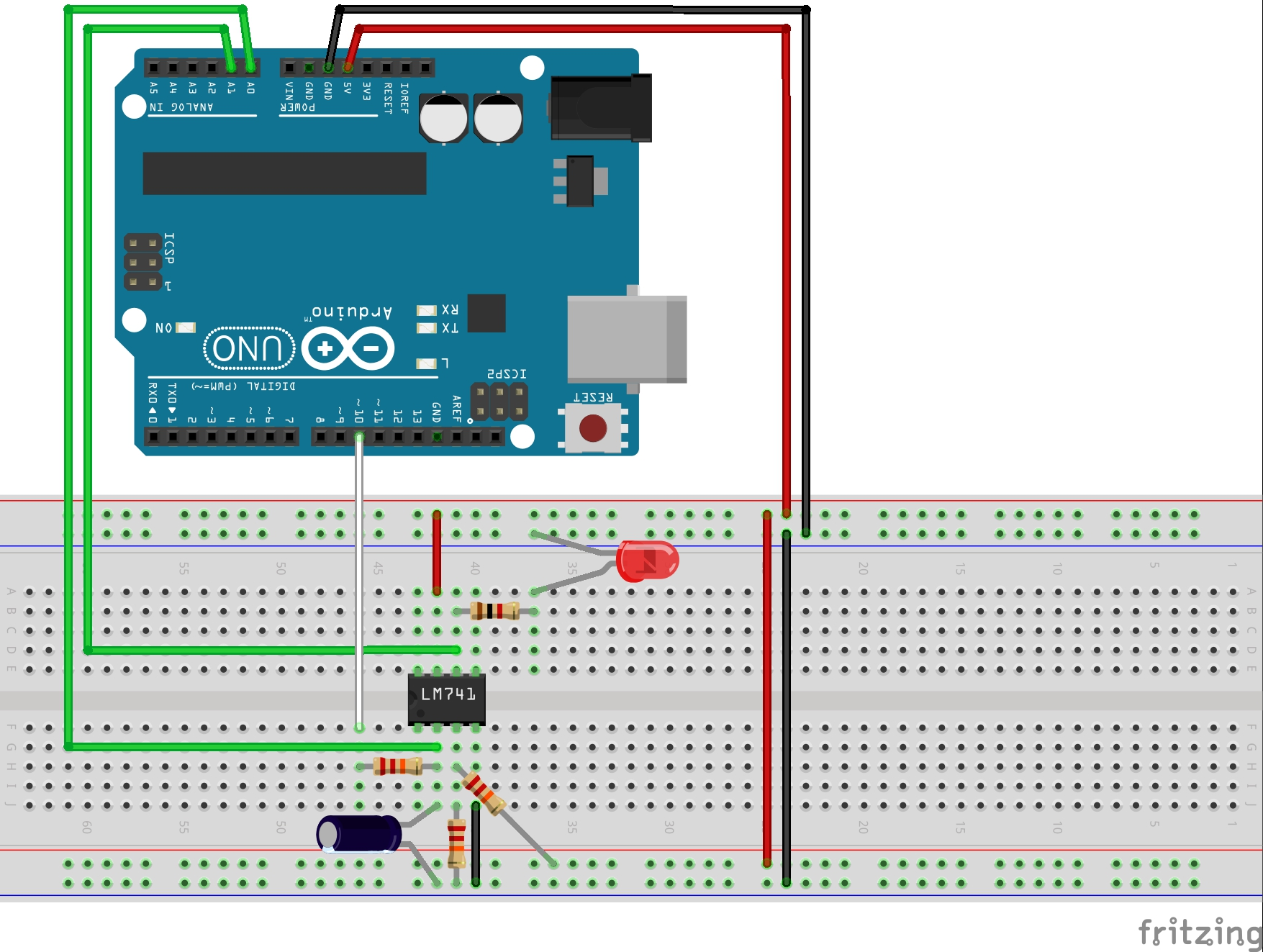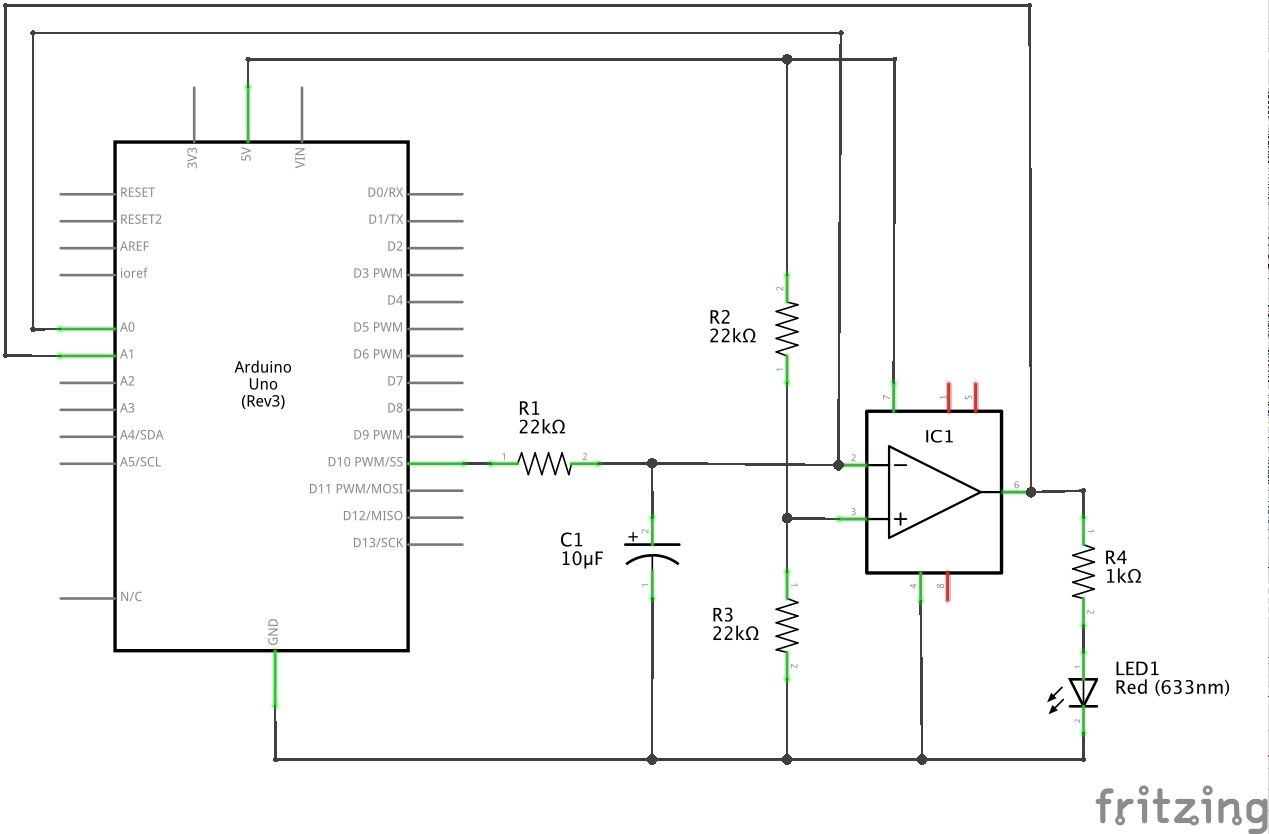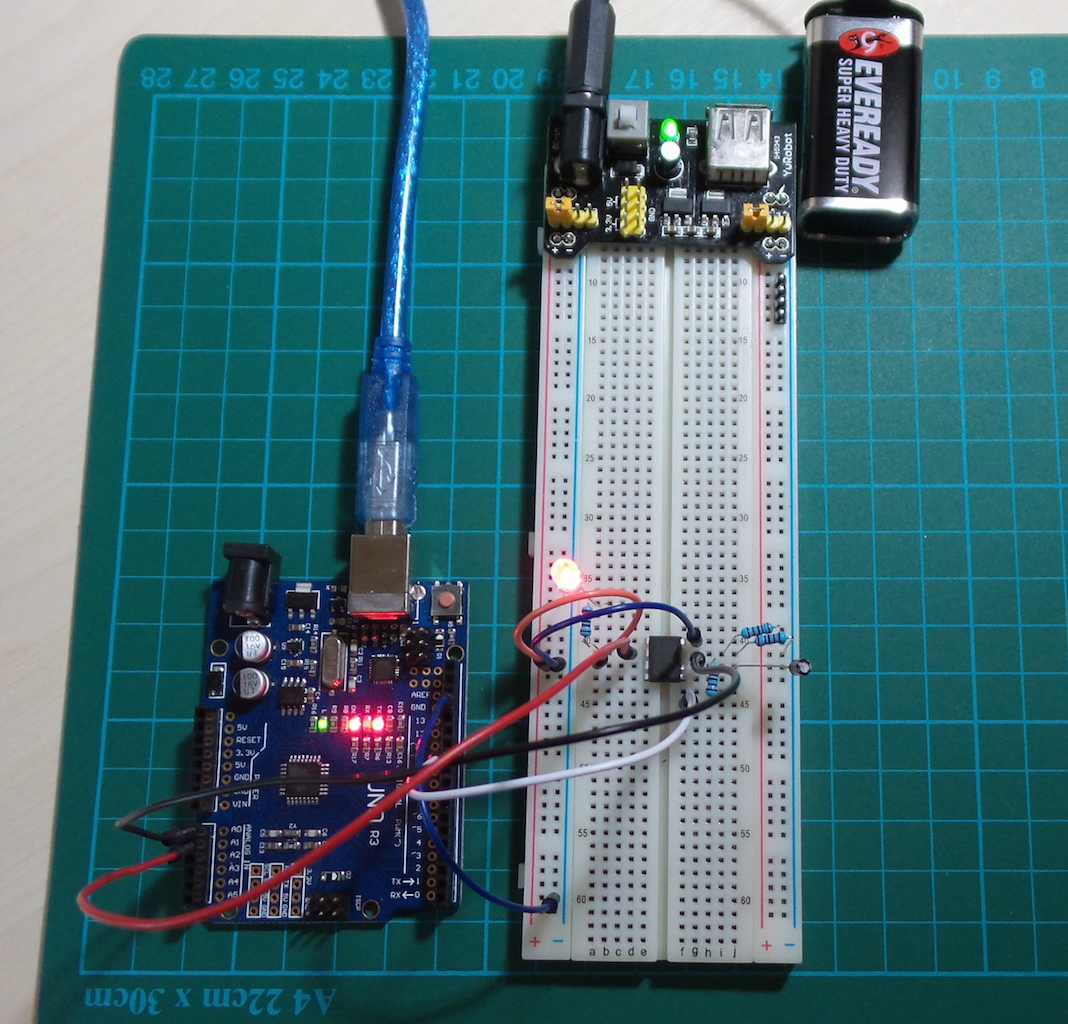# #052 Comparator741 - LM741 OpAmp Comparator Behaviour

Uses an Arduino to test LM741 comparator behaviour and plot the results with processing

### Testing Characteristic Comparator Behaviour

Before hooking up the Arduino and driving the LM741 with PWM, I used a test circuit with variable resistors to verify - see a picture of the build.

The 741 is powered with:

``````V+ : 5.00V
V- : 0.00V
``````

Here are a couple of measurements:

IN- IN+ Vout
2.52 2.41 1.58
3.23 2.41 1.58
2.21 2.41 4.14
2.21 2.06 1.58
2.21 1.50 1.58

This demonstrates that when the inverting input voltage is below the non-inverting input, Vout goes high and vice versa. High and low output voltages are measure at 4.14 and 1.58V respectively, which is to be expected since the 741 is not capable of rail-to-rail output.

### Driving with PWM

The objective of the Arduino sketch is to drive the non-inverting to various voltages under automation, with the inverting reference voltage set to Vcc/2 (2.5V) with a simple voltage divider.

Since the Arduino’s analogWrite is actually a PWM signal, it is necessary to peform a PWM-to-analogue conversion, otherwise the 741 would simply oscillate according to the PWM signal.

The circuit uses a simple RC low-pass filter to perform the conversion. This introduces a time constant to the non-inverting voltage that inhibits instantaneous change.

For an Arduino Uno, the frequency of the PWM signal on pin 10 is approximately 490 Hz. The 2.5V mid-point is generated with 50% duty cycle PWM pulse, and therefore the signal is low for ~1ms then high for ~1ms.

So we need an RC time constant (𝛕) that will sufficiently bridge the 1ms “gap”. The trade-off is that reducing the voltage ripple by increasing the time constant will also slow down the filter response to voltage changes.

In this case I have gone for “extreme dampening” with R=22kΩ and C=10μF, resulting in a time constant of 220ms. As can be seen in the trace below, this results in a very smooth inverting voltage source and consequently distinct output changes for the OpAmp comparator. But there is also an appreciable lag behind that actual PWM output change.

### Measured Results

The comparator behaviour is demonstrated in the following trace with R=22kΩ and C=10μF.

• upper trace it the OpAmp output voltage
• middle trace is the inverting input voltage
• lower trace is the actual PWM output valuePlotNValues (a simple Processing sketch) reads the data from the serial port and plots the values over time, with some coloration effects thrown in for good measure. In other words, we’re using Arduino and Processing as a basic oscilloscope .. and it kind of works, mainly because the frequency is so low.

### Construction

The Arduino acts as a controller and measurement device in this circuit.

The circuit is powered directly from the Arduino 5V pin, although it could also be powered by an independent 5V supply. Note that if the external power supply is over 5V, the analog inputs should be scaled back with a voltage divider to avoid overloading Arduino pins.